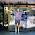Thursday, November 3, 2011

Somebody bet on the Bayes

In last week's post I wrote solutions to some of my favorite Bayes's Theorem problems, and posed this new problem:
If you meet a man with (naturally) red hair, what is the probability that neither of his parents has red hair?
Hints: About 2% of the world population has red hair.  You can assume that the alleles for red hair are purely recessive.  Also, you can assume that the Red Hair Extinction theory is false, so you can apply the Hardy–Weinberg principle.
Given the prevalence of red hair, we know what fraction of the population is homozygous recessive.  To solve the problem, we also need to know how many are heterozygous and homozygous dominant.

I'll use a to represent the recessive allele (or alleles) for red hair, and A for the dominant alleles that code for other colors.  p is the prevalence of a and q is the prevalence of A, so p+q = 1.

If these prevalences are not changing over time, and they don't affect people's mating decisions, we can invoke the Hardy-Weinberg principle to get:

P(AA) = prevalence of homozygous dominant = q**2
P(Aa) = prevalence of heterozygous = 2 * p * q
P(aa) = prevalence of homozygous recessive = p**2

And P(AA) + P(Aa) + P(aa) = 1.

Given (aa), we can compute p, q and:

P(Aa) = 0.243
P(AA) = 0.737

Now if a child has red hair, both parents have at least one recessive allele, so the possible combinations are (Aa Aa), (Aa aa), and (aa aa).  If we assume, again, that mating decisions are not based on hair color, we can get the prevalence of each parental pair:

P(Aa Aa) = Aa**2
P(Aa aa) = 2 Aa aa
P(aa aa) = aa**2

And we can use those as the priors.  The evidence is

E: the child has red hair

To get the likelihoods, we apply Mendelian genetics:

P(E | Aa Aa) = 0.25
P(E | Aa aa) = 0.5
P(E | aa aa) = 1.0

Finally, applying Bayes's Theorem, we have

P(Aa Aa | E) = P(Aa Aa) P(E | Aa Aa) / P(E)

And the answer is 0.737.  With a little algebra we can show that

P(Aa Aa | E) = P(AA)

That is, the probability that neither parent has red hair is exactly the fraction of the population that is homozygous dominant.

Almost 75% of red-haired people come from parents with non-red hair, which might explain why a "red haired child" is a metaphor for a child that doesn't resemble his parents. In the expression, "beat like a red haired step child," some of the humor (for people who find child abuse funny) comes from the suggestion that the parentage of a red haired child is suspect.

But why should red hair be funnier than blue eyes or blonde hair?  It turns out that we can answer this question mathematically. If the prevalence of red hair were higher, say 10%, most red haired people would have at least one red-haired parent, and that would be less funny.

In general, as the prevalence of the recessive phenotype increases, the potential for amusing insinuations of infidelity decreases; near 0 it drops off steeply, as shown in this figure:

Since I believe I am the first person to quantify this effect, I humbly submit that it should be called "Downey's inverse law of mailman jokes."

For more fun with probability, see Chapter 5 of my book, Think Stats, which you can read here, or buy here.

-----

If you don't get the title of this post, it is a play on "Somebody bet on the bay," a lyric from the minstrel song "Camptown Races."  A bay is a horse with a reddish-brown coat, so I thought it was a pretty good fit.

1.I found it a little easier to analyze this situation by realizing that you can calculate the probability of each parent's readheadedness separately. The prior probabilities relating to the two parents are independent, and the evidence "factors" into a statement just about the mother (mother passed on "a" to child) and a statement just about the father (father passed on "a" to child). So the posterior probability for the various genetic makeups of the mother and father are independent, and you can calculate them one at a time.

Consider just the mother. The prior probabilities for her genetic makeup are what you said:

p(AA)=q^2
P(Aa)=2pq
P(aa)=p^2

The evidence E is that the mother passed on a to her child.

P(E | AA) = 0
P(E | Aa) = 1/2
P(E | aa) = 1

Turning the Bayes's theorem crank, we get

P(AA | E) = 0 (of course)
P(Aa | E) = q
P(aa | E) = p

So the probability that the mother didn't have red hair is q. The same reasoning works for the father, so the probability that neither had red hair is q^2.

2.@Ted: very nice. Thanks!

When I did the algebra and everything worked out so neatly, I should have recognized that a symptom that there is an easier way to get there.

3.That's exactly what happened to me. I did it the way you did first then decided based on the relatively simple answer that there must be another way.